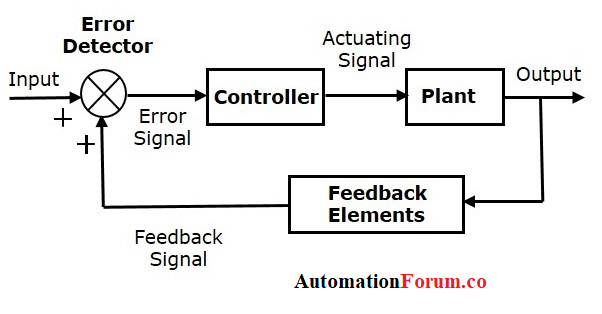# Basics of feedback systemThere are several techniques used for the process control system that are useful in the analysis and design of the modern process control system. The feedback control system is a type used in this type of control system.

### Feedback control system:

In the feedback, the output of the control system is measured and compared to the input reference signal. The deviation (error) between the two is used by the controller that will send the output so that the system error is zero.

A feedback system is one in which a sample of the output signal is taken and then sent to the input to form an error signal that drives the system.

In a Feedback System, all or part of the output signal either positive or negative is fed back to the input.

Feedback Systems process signals and as such are signal processors. The processing part of a feedback system may be electrical or electronic, ranging from a very simple to a highly complex circuits.

### Why feedback control systems are used?

• The characteristics of the circuit, such as the gain and response of the system, can be controlled with precision.
• Circuit characteristics can be made independent of operating conditions such as supply voltages or temperature variations.
• The distortion of the signal due to the non-linear nature of the components used can be considerably reduced.
• The frequency response, gain and bandwidth of a circuit or system can be easily controlled within tight limits.

There are just two main types of feedback control namely: Negative Feedback and Positive Feedback.

#### Positive feedback system:

Positive feedback control of the op-amp is achieved by applying a small part of the output voltage signal at Vout back to the non-inverting ( + ) input terminal via the feedback resistor, RF.

If the input voltage Vin is positive, the operational amplifier amplifies this positive signal and the output becomes more positive. Part of this output voltage is returned to the input by the feedback network. Therefore, the input voltage becomes more positive, causing an even higher output voltage and so on. Finally, the output saturates in its positive supply lane.

#### Negative feedback system

In a negative feedback control system, the set point and output values are subtracted from each other since the feedback is out of phase with the original input. The effect of negative (or degenerative) feedback is to reduce the gain.

If the input voltage Vin is positive, the operational amplifier amplifies this positive signal, but because it is connected to the inverting input of the amplifier, the output becomes more negative. Part of this output voltage is returned to the input by the feedback network of Rf. Therefore, the input voltage is reduced by the negative feedback signal, which causes an even smaller output voltage

### Advantage of feedback control system:

• It is very simple technique that compensates for all disturbances
• The controller adjusts the controlled variable (affected by the disturbance) with the set point
• The feedback control circuit does not worry about the disturbance that enters the process

### Disadvantage of feedback control system

• It does not take corrective measures until after the disturbance has altered the process and generated an error signal.
• The feedback controllers continue changing its output until measurement and set point are in agreement.

Scroll to Top